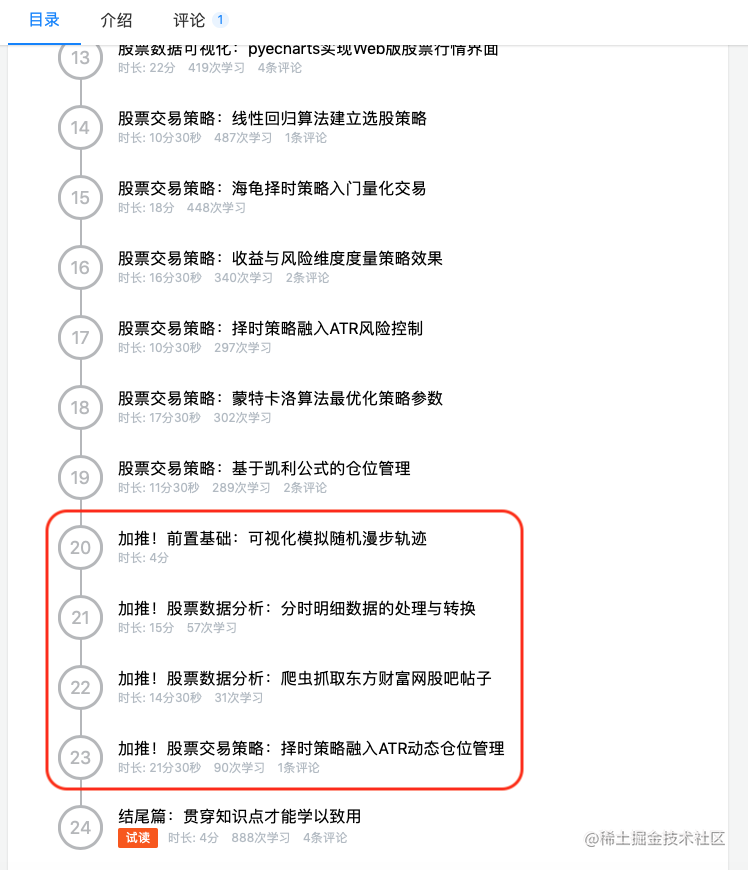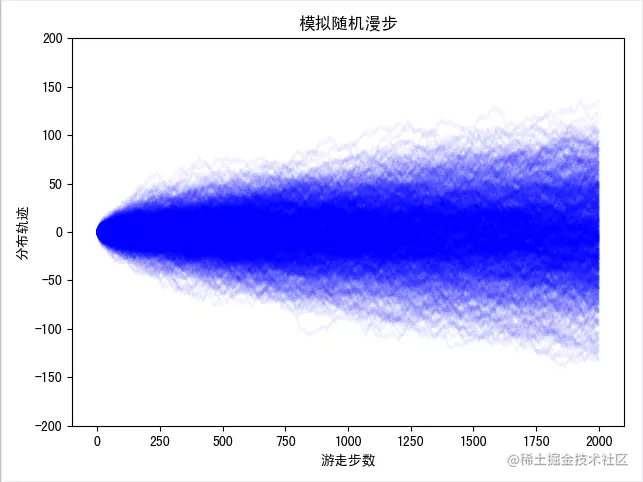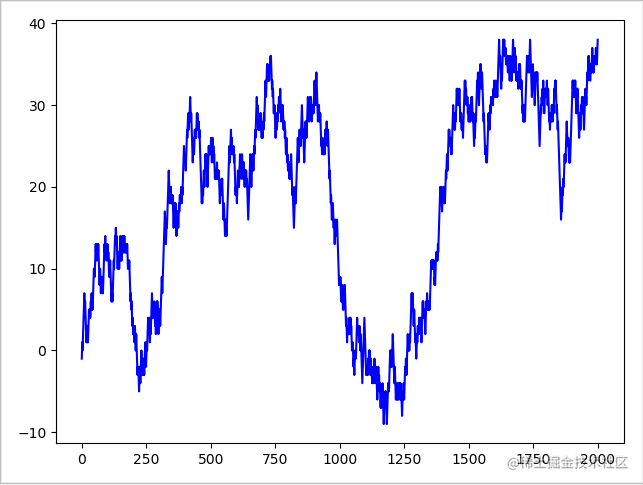Python量化交易进阶讲堂-可视化随机漫步轨迹前言生成随机漫步数组

• 返回随机整数，范围区间为[low,high），包含low，不包含high
• 参数：low为最小值，high为最大值，size为数组维度大小，dtype为数据类型，默认的数据类型是np.int
• high没有填写时，默认生成随机数的范围是[0，low]
print("np.random.randint：\n {}".format(np.random.randint(1,size=5)))# 返回[0,1)之间的整数，所以只有0
"""
np.random.randint：
[0 0 0 0 0]
"""
print("np.random.randint：\n {}".format(np.random.randint(1,5)))# 返回1个[1,5)时间的随机整数
"""
np.random.randint：
2
"""
print("np.random.randint：\n {}".format(np.random.randint(-5,5,size=(2,2))))
"""
np.random.randint：
[[-5 -3]
[ 2 -3]]
"""

可视化随机漫步轨迹

draws = np.random.randint(0, 2, size=nsteps)
steps = np.where(draws > 0, 1, -1)
walk = steps.cumsum()_ = [plt.plot(np.arange(2000), random_walk(nsteps=2000), c='b', alpha=0.05) for _ in np.arange(0,1000)]